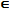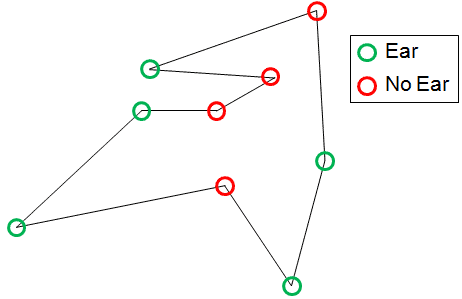Triangulation of Simple Polygons with Kong's AlgorithmDownload Applet including Source (57kb)

Welcome to part III of my Polygon Algorithm Series.
I am showing you my implementation of Kong's Algorithm to divide a simple polygon into triangles which is called triangulation. A simple polygon is a polygon which is has no holes, thus its edges must not touch or overlap, but it does not have to be convex. Kong's Algorithm is quite simple as it goes along all points of the polygon and cuts off convex vertices (thus generate a triangle) as long as there are more than 4 vertices left.

Given: Simple Polygon P1, P2, ..., Pn with n vertices
Goal: A set of triangles (Pi, Pj, Pk) with i,j,k{1,..,n} which forms a complete, non-overlapping triangulation of the polygon

Definition Ear: An Ear is a convex vertice of the polygon (Pi, Pi+1, Pi+2), that means the segment (Pi,Pi+2) is completely inside the polygon and is not touched/cut by any other edge of the polygon.The algorithm goes along all edges and checks if 3 neighboring points Pi, Pi+1, Pi+2 build an ear.
If Yes: Build triangle Pi, Pi+1, Pi+2, remove Pi+1 from the polygon, go one vertice back and proceed.
If Not: Proceed with checking Pi+1, Pi+2, Pi+3.
Terminate if only 3 points are left, those build the last triangle.

The algorithm is based on the fact that each simple polygon contains at least 2 Ears. The proof is not given here, but is easy to do with induction. To speed up the algorithm, especially the ear detection, at first all non-convex vertices are saved in a data structure for easy access - this works as no additional ears are created during algorithm execution.The picture above shows an example of the Kong Algorithm. The blue triangle is the one currently to be observed. The red ones are the triangles already cutted away and do not longer belong to the actual polygon. In step 5, the three points do not form an ear so the algorithm proceed with the next three points.
Although the algorithm is really simple, there are some minor issues in implementation. In Ear Detection, each vertice of the polygon is checked for convexity - therefore the direction (in which the user gave the consecutive vertices) must be known - that is if the user 'painted' the polygon clockwise or counter-clockwise. This can be accomplished by determining the vertice which has the smallest x-coordinate (if there are several with the same smallest x-coordinate, choose the one which has the biggest y-coordinate) and checking both edges which have this vertice in common if there is a right turn or a left turn. Convexity I have already discussed in Part I of my polygon algorithm series. This is enough to detect ears and the actual algorithm is quite simple, so just refer to the source code :-)

Pseudocode:

 // Precondition: R contains all non-convex points of polygon BOOL isEar(Vertice P-1, Vertice P, Vertice P1) begin     If R is empty         return False     else         if x is convex         then             if InsideAreaOfTriangle(P-1, P, P1) contains no point of R                 return true            else                 return false         else             return false end // Preconditions: // Q contains all points/vertices of the polygon void DoKong() begin     while |Q| > 3         if isEar(P-1, P, P1) then             addTriangle(P-1, P, P1)       // e.g. to a list             remove P from Q             P = P-1;     else         P = P1; end

Sunshine, February 2k9.

This site is part of Sunshine's Homepage